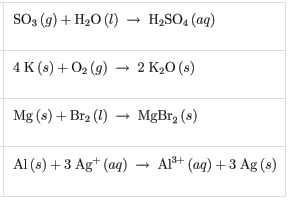# Problem: 1. A beaker of nitric acid is neutralized with calcium hydroxide. Express your answer as a chemical equation. Identify all of the phases in your answer.a. Write a balanced molecular equation for this reaction.b. Write a net ionic equation for this reaction.2. Which of the following reactions are redox reactions?

###### FREE Expert Solution
79% (380 ratings)###### Problem Details

1. A beaker of nitric acid is neutralized with calcium hydroxide. Express your answer as a chemical equation. Identify all of the phases in your answer.

a. Write a balanced molecular equation for this reaction.

b. Write a net ionic equation for this reaction.

2. Which of the following reactions are redox reactions?